Next: General Properties of Stress Up: Mathematical Models of Fluid Previous: What is a Fluid?

# Volume and Surface Forces

Generally speaking, fluids are acted upon by two distinct types of force. The first type is long range in nature--that is, such that it decreases relatively slowly with increasing distance between interacting elements--and is capable of completely penetrating into the interior of a fluid. Gravity is an obvious example of a long-range force. One consequence of the relatively slow variation of long-range forces with position is that they act equally on all of the fluid contained within a sufficiently small volume element. In this situation, the net force acting on the element becomes directly proportional to its volume. For this reason, long-range forces are often called volume forces. In the following, we shall write the total volume force acting at timeon the fluid contained within a small volume element of magnitude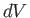, centered on a fixed point whose position vector is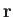, as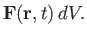(1.1)

The second type of force is short range in nature, and is most conveniently modeled as momentum transport within the fluid. Such transport is generally due to a combination of the mutual forces exerted by contiguous molecules, and momentum fluxes caused by relative molecular motion. Suppose that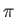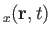is the net flux density of-directed fluid momentum due to short-range forces at positionand time. In other words, suppose that, at positionand time, as a direct consequence of short-range forces,-momentum is flowing at the rate of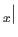newton-seconds per meter squared per second in the direction of vector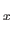. Consider an infinitesimal plane surface element,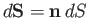, located at point. Here,is the area of the element, and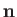its unit normal. (See Section A.7.) The fluid which lies on that side of the element toward whichpoints is said to lie on its positive side, and vice versa. The net flux of-momentum across the element (in the direction of) is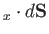newtons, which implies (from Newton's second law of motion) that the fluid on the positive side of the surface element experiences a forcein the-direction due to short-range interaction with the fluid on the negative side. According to Newton's third law of motion, the fluid on the negative side of the surface experiences a force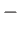in the-direction due to interaction with the fluid on the positive side. Short-range forces are often called surface forces, because they are directly proportional to the area of the surface element across which they act. Let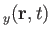and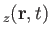be the net flux density of- and-momentum, respectively, at positionand time. By a straightforward extension of previous argument, the net surface force exerted by the fluid on the positive side of some planar surface element,, on the fluid on its negative side is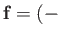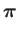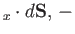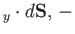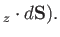(1.2)

In tensor notation (see Appendix B), the previous equation can be written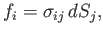(1.3)

where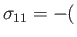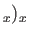,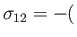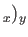,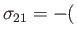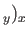, et cetera. (Note that, because the subscript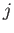is repeated in the previous equation, it is assumed to be summed fromto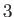. Hence,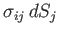is shorthand for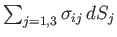.) Here, the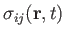are termed the local stresses in the fluid at positionand time, and have units of force per unit area. Moreover, the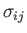are the components of a second-order tensor (see Appendix B) known as the stress tensor. [This follows because the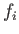are the components of a first-order tensor (as all forces are proper vectors), and the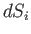are the components of an arbitrary first-order tensor (as surface elements are also proper vectors--see Section A.7--and Equation (1.3) holds for surface elements whose normals point in any direction), so application of the quotient rule (see Section B.3) to Equation (1.3) reveals that thetransform under rotation of the coordinate axes as the components of a second-order tensor.] We can interpretas the-component of the force per unit area exerted, at positionand time, across a plane surface element normal to the-direction. The three diagonal components ofare termed normal stresses, because each of them gives the normal component of the force per unit area acting across a plane surface element parallel to one of the Cartesian coordinate planes. The six non-diagonal components are termed shear stresses, because they drive shearing motion in which parallel layers of fluid slide relative to one another.Next: General Properties of Stress Up: Mathematical Models of Fluid Previous: What is a Fluid?
Richard Fitzpatrick 2016-03-31